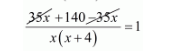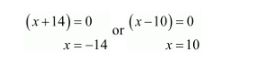# A piece of cloth costs Rs. 35.

Question:

A piece of cloth costs Rs. 35. If the piece were 4 m longer and each meter costs Rs. one less, the cost would remain unchanged. How long is the piece?

Solution:

Let the length of the piece be x metres.

Then, rate per metre $=\frac{35}{x}$

According to question, new length $=(x+4)$ meters.

Since the cost remain same. Therefore, new rate per metre $=\frac{35}{x+4}$

It is given that

$\frac{35}{x}-\frac{35}{x+4}=1$

$\frac{35(x+4)-35 x}{x(x+4)}=1$$\frac{140}{x(x+4)}=1$

$x^{2}+4 x=140$

$x^{2}+4 x-140=0$

$x^{2}+14 x-10 x-140=0$

$x(x+14)-10(x+14)=0$

$(x+14)(x-10)=0$Because $x$ cannot be negative.

Thus, $x=10$ is the require solution.

Therefore, the length of the piece be $x=10$ metres